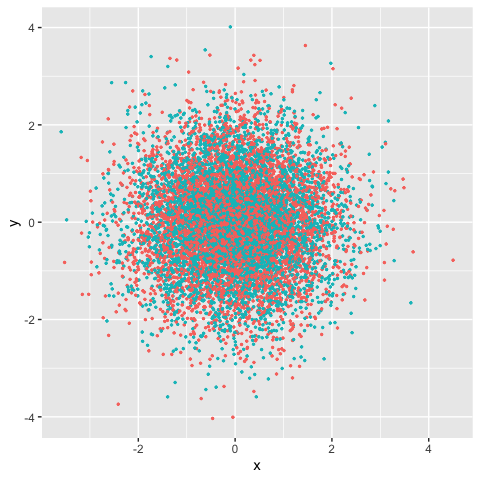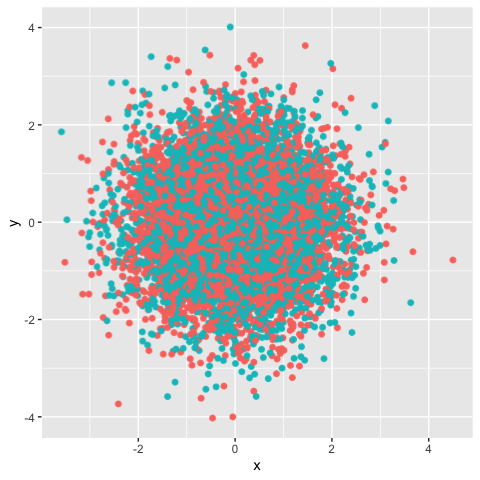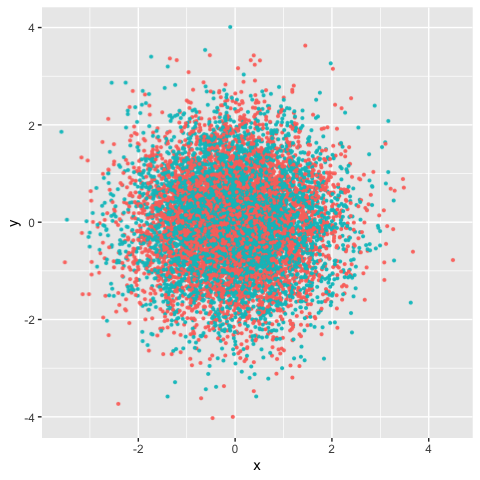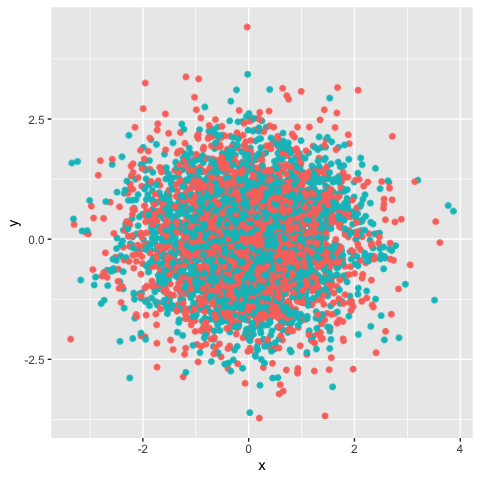Legacy (outdated) wrapper functions

For historic reasons, ggrastr used to be solely composed of the following functions:

• geom_point_rast: raster scatter plots
• geom_jitter_rast: raster jittered scatter plots
• geom_boxplot_jitter: boxplots that allows to jitter and rasterize outlier points
• geom_tile_rast: raster heatmap
• geom_beeswarm_rast: raster bee swarm plots
• geom_quasirandom_rast: raster quasirandom scatter plot

However, we strongly encourage users to use the rasterise() function instead. For posterity’s sake, we have only included the old vignettes here for the reference of users, along with the equivalent functions using rasterise().

Points: Rasterize scatter plots with geom_point_rast()

Sometimes you need to publish a figure in a vector format:

library(ggplot2)
library(ggrastr)
points_num <- 10000
df <- data.frame(x=rnorm(points_num), y=rnorm(points_num), c=as.factor(1:points_num %% 2))
gg <- ggplot(df, aes(x=x, y=y, color=c)) + scale_color_discrete(guide="none")
gg_vec <- gg + geom_point(size=0.5)
print(gg_vec)But in other cases, your figure contains thousands of points, e.g. try points_num <- 500000 in the example above, and you will notice the performance issues—it takes significantly longer to render the plot.

In this case, a reasonable solution would be to rasterize the plot. But the problem is that all text becomes rasterized as well.

Raster layers with ggrastr were developed to prevent such a situation, using `rasterized

gg_rasterized <- gg + rasterise(geom_point(), dpi = 300, scale = 1)
print(gg_rasterized)The legacy function used in older versions of ggrastr was geom_point_rast():

gg_rast <- gg + geom_point_rast(size=0.5)
print(gg_rast)The plots look the same, but the difference in size can be seen when they are exported to pdfs. Unfortunately, there is a longer rendering time to produce such plots:

PrintFileSize <- function(gg, name) {
invisible(ggsave('tmp.pdf', gg, width=4, height=4))
cat(name, ': ', file.info('tmp.pdf')\$size / 1024, ' Kb.\n', sep = '')
}
PrintFileSize(gg_rast, 'Raster')
#> Raster: 291.5576 Kb.
PrintFileSize(gg_vec, 'Vector')
#> Vector: 556.1484 Kb.

As expected, the difference becomes larger with growth of number of points:

points_num <- 1000000
df <- data.frame(x=rnorm(points_num), y=rnorm(points_num), c=as.factor(1:points_num %% 2))
gg <- ggplot(df, aes(x=x, y=y, color=c)) + scale_color_discrete(guide="none")
gg_vec <- gg + geom_point(size=0.5)
gg_rast <- gg + geom_point_rast(size=0.5)
PrintFileSize(gg_rast, 'Raster')
#> Raster: 358.6611 Kb.
PrintFileSize(gg_vec, 'Vector')
#> Vector: 54786.11 Kb.

Jitter: Rasterize jittered scatter plots with geom_jitter_rast()

Users may also opt to create rasterized scatter plots with jitter:

library(ggplot2)
library(ggrastr)
points_num <- 5000
df <- data.frame(x=rnorm(points_num), y=rnorm(points_num), c=as.factor(1:points_num %% 2))
gg <- ggplot(df, aes(x=x, y=y, color=c)) + scale_color_discrete(guide="none")
gg_jitter_rast <- gg + rasterise(geom_jitter(), dpi = 300, scale = 1)
print(gg_jitter_rast)The legacy wrapper geom_jitter_rast() used the following syntax:

library(ggplot2)
library(ggrastr)

points_num <- 5000
df <- data.frame(x=rnorm(points_num), y=rnorm(points_num), c=as.factor(1:points_num %% 2))
gg <- ggplot(df, aes(x=x, y=y, color=c)) + scale_color_discrete(guide=FALSE)

gg_jitter_rast <- gg + geom_jitter_rast(raster.dpi=600)
print(gg_jitter_rast)
#> Warning: It is deprecated to specify `guide = FALSE` to remove a guide. Please
#> use `guide = "none"` instead.